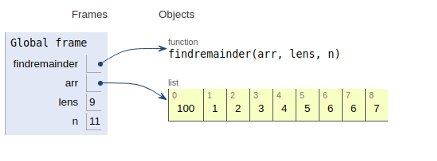# Python Program for Find reminder of array multiplication divided by n

## Problem statement

Given multiple numbers and a number input n, we need to print the remainder after multiplying all the number divisible by n.

## Approach

• First, compute the remainder like arr[i] % n. Then multiply this remainder with the current result.

• After multiplication, again take the same remainder to avoid overflow. This is in accordance with distributive properties of modular arithmetic.

( a * b) % c = ( ( a % c ) * ( b % c ) ) % c

## Example

Live Demo

def findremainder(arr, lens, n):
mul = 1
# find the individual remainder
for i in range(lens):
mul = (mul * (arr[i] % n)) % n
return mul % n
# Driven code
arr = [100,1,2,3,4,5,6,6,7]
lens = len(arr)
n = 11
print( findremainder(arr, lens, n))

## Output

1

All the variables are declared in the global frame as shown in the figure given below## Conclusion

In this article, we learned about the approach to Find reminder of array multiplication divided by n